## Title: plot-3d-MVNormal-1.R
## Author: Paul Johnson <pauljohn at ku.edu>
## Date posted: 2011->
## Description. A little file of test cases

## 3d plots of 2 dimensional normal distribution
## Paul Johnson < pauljohn@ku.edu >

library(mvtnorm)

library(help=mvtnorm)

N <- 100

x <- seq(-3,3, length=N)
y <- seq(-3,3,length=N)
z <- matrix(0, N, N)
for (i in 1:N) for (j in 1:N) {
z[i,j] <- dmvnorm(c(x[i],y[j]), c(0,0),
matrix(c(1,0.5,0.5,1),2,2)) }
persp(x,y,z)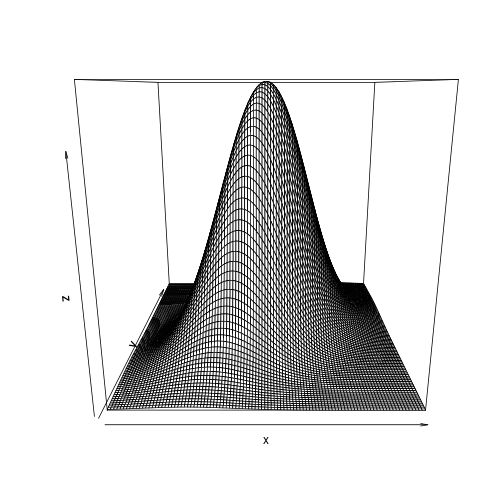# using "outer" makes previous for loop unnecessary,
# but it requires additional work in arranging everything

meanX <- c( 1 , 1 )

varX <- matrix ( c( 1.1, 1.4, 1.4, 5.3), nrow=2 , byrow = TRUE)

# Note this gives a Var-Cov matrix

#             1.1      0.8
#             0.8      2.3
#

x1 <- seq( meanX-3*varX[1,1], meanX+3*varX[1,1], length.out=N)
x2 <- seq(  meanX-3*varX[2,2], meanX+3*varX[2,2] , length.out=N)

myProbX <- function(x1, x2){
dmvnorm( cbind(x1,x2), mean = meanX, sigma= varX)
}

probX <- outer(x1,x2, FUN="myProbX")

persp(x1,x2, probX, theta = 140, phi = 10, zlim=c(0,0.5), col = "pink")# theta turns the X,Y plane counterclockwise, default = 0
# phi moves you up above the graph, to look down, default = 15

persp(x1,x2, probX, theta = 50, phi = 20, zlim=c(0,0.5), col = "pink",ticktype="detailed")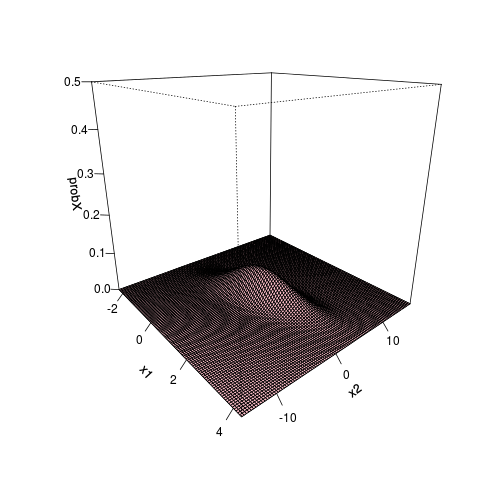persp(x1,x2, probX, theta = 140, phi = 10, zlim=c(0,0.1), col = "pink")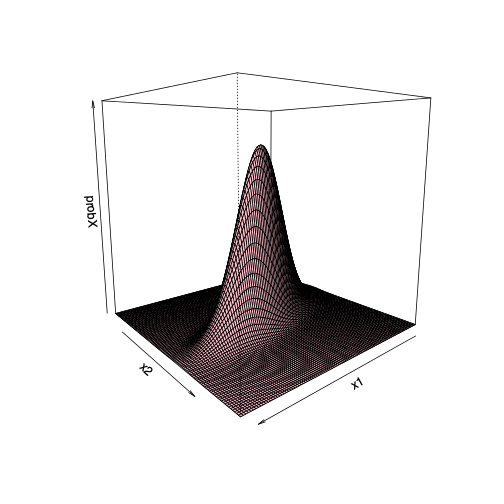persp(x1,x2, probX, theta = 40, phi = 10, zlim=c(0,0.1), col = "pink")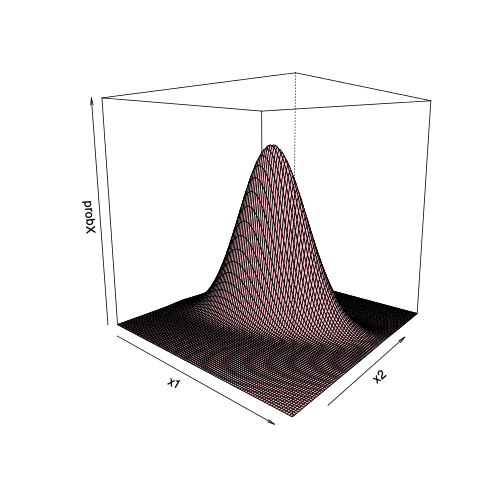persp(x1,x2, probX, theta = 66, phi = 10, zlim=c(0,0.1), col = "pink")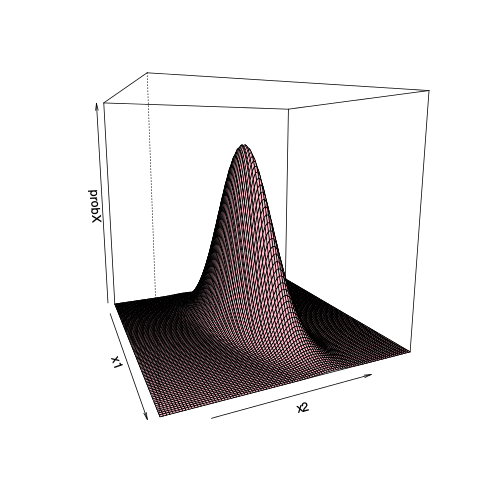persp(x1,x2, probX, theta = 140, phi = 20, zlim=c(0,0.1), col = "pink", ltheta=144, lphi=100, shade=0.4, axes=T)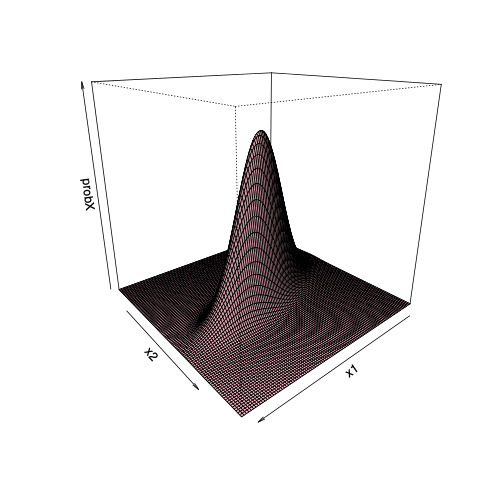persp(x1,x2, probX, theta = 140, phi = 20, zlim=c(0,0.1), border=NA)persp(x1,x2, probX, theta = 140, phi = 20, zlim=c(0,0.1), border=NA, shade=2.1)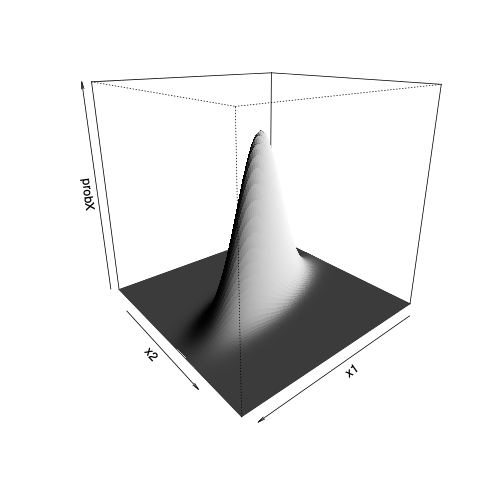persp(x1,x2, probX, theta = 140, phi = 20, zlim=c(0,0.1), border=NA, shade=1, ltheta=20, lphi=20)